Question

1. A centrifugal pump performance data are shown in the following table for water. Pump speed-250...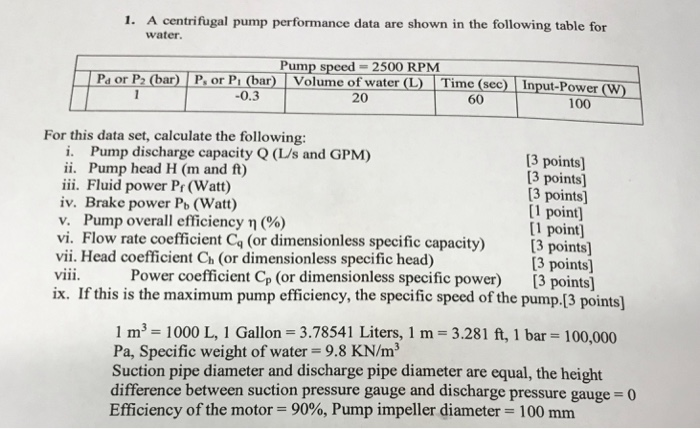1. A centrifugal pump performance data are shown in the following table for water. Pump speed-2500 RPM Pa or P2 (bar) P. or Pi (bar) Volume of water(L Time (sec) Input-Power (W) -0.3 20 60 100 For this data set, calculate the following i. Pump discharge capacity Q (LUs and GPM) ii. Pump head H (m and ft) iii. Fluid power Pr (Watt) iv. Brake power Pb (Watt) v. Pump overall efficiency η (%) vi. Flow rate coefficient Cq (or dimensionless specific capacity vii. Head coefficient Ch (or dimensionless specific head) vii. Power coefficient Cp (or dimensionless specific power) [3 points] ix. If this is the maximum pump efficiency, the specific speed of the pump.[3 points] 3 points] 3 points] 3 points] [1 point] 1 point] [3 points 3 points] 1 m3 1000 L, 1 Gallon 3.78541 Liters, 1 m 3.281 ft, 1 bar 100,000 Pa, Specific weight of water- 9.8 KN/m3 Suction pipe diameter and discharge pipe diameter are equal, the height difference between suction pressure gauge and discharge pressure gauge 0 Efficiency of the motor 90%, Pump impeller diameter-100 mm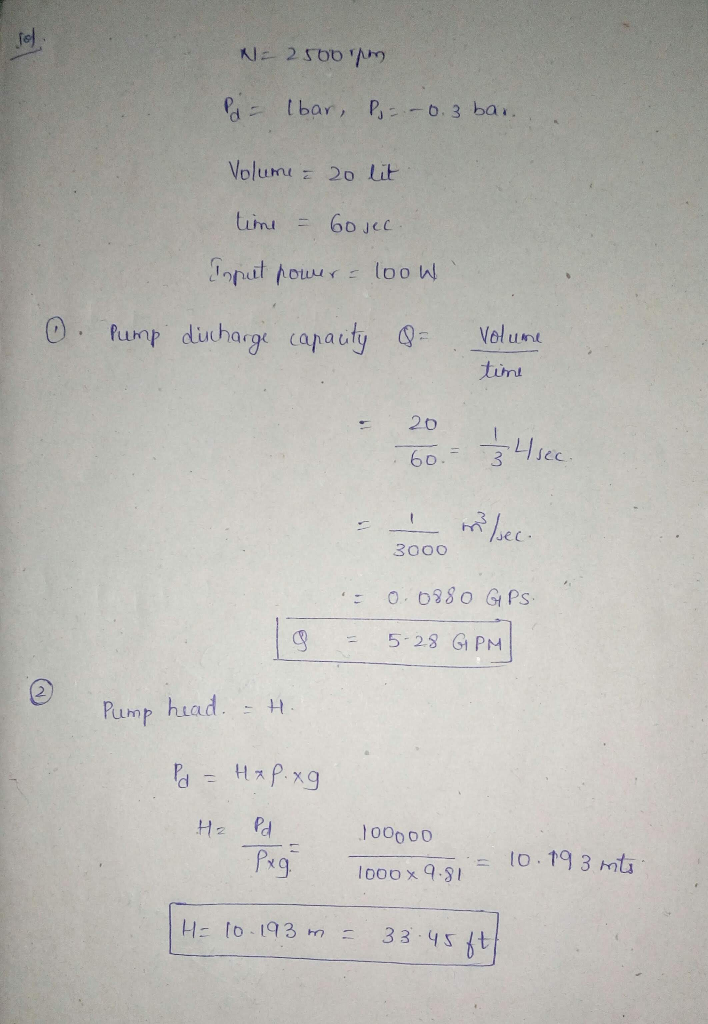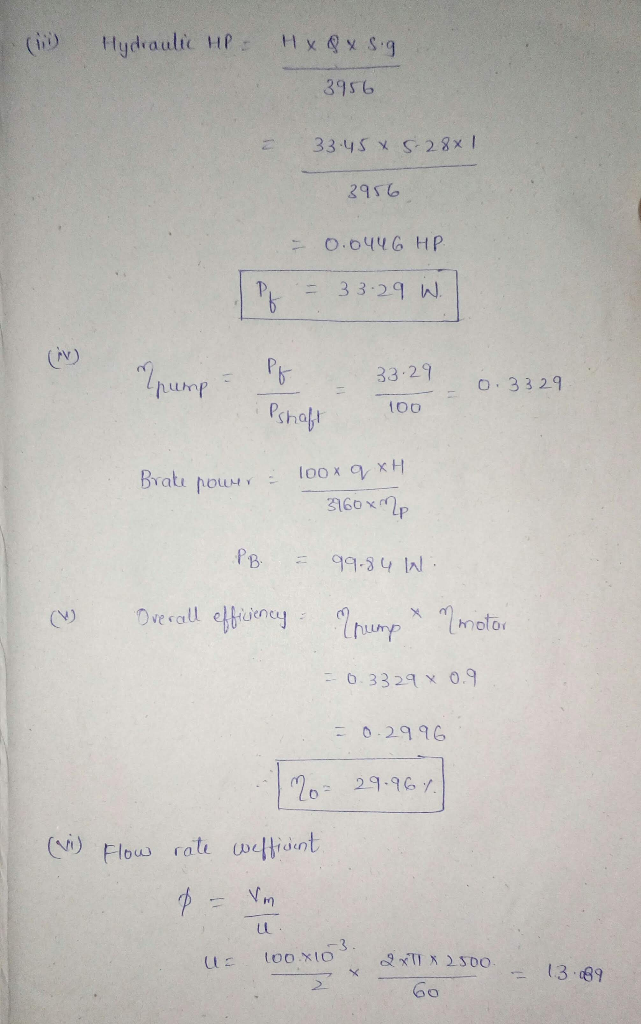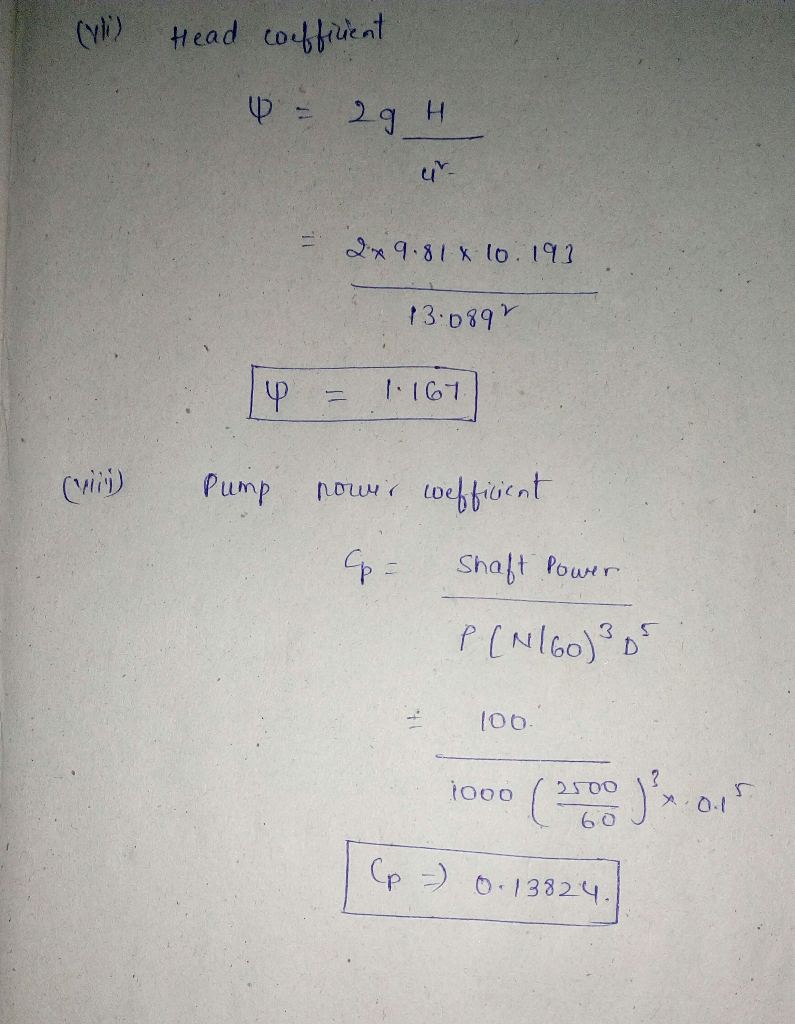Earn Coins

Coins can be redeemed for fabulous gifts.

Similar Homework Help Questions
• 3. A centrifugal pump performance data are shown in the following table for water. Pump speed...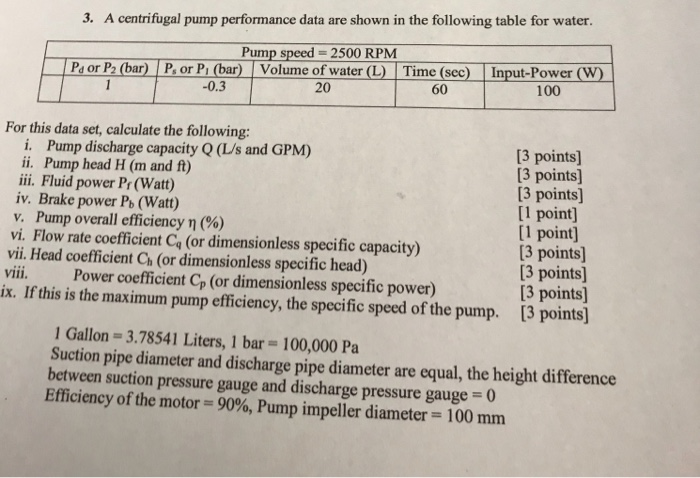3. A centrifugal pump performance data are shown in the following table for water. Pump speed 2500 RPM Pe or P2 (bar) P or Pi (bar) Volume of water (L) Time (sec) Input-Power (W -0.3 20 60 100 For this data set, calculate the following: i. Pump discharge capacity Q (L/s and GPM) ii. Pump head H (m and f) iii. Fluid power Pr(Watt) iv. Brake power Pb (Watt) v. Pump overall efficiency η (%) vi. Flow rate coefficient Ca...

• Q4. A pump draws water from a large reservoir and delivers it to another reservoir whose water surface is 10 m higher than the pump centreline and that the pump is 1.5 meter above the suction reservo...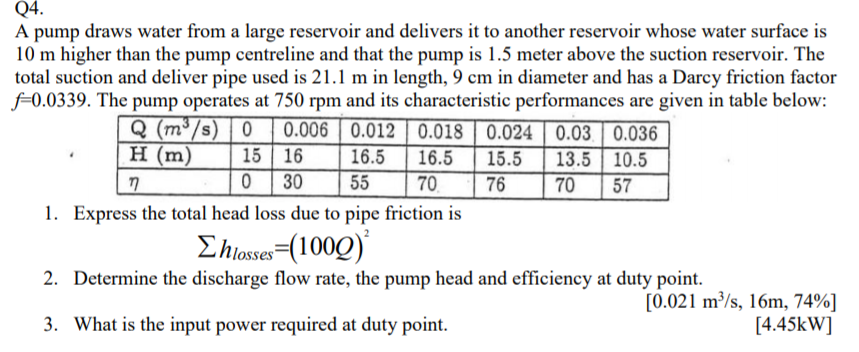Q4. A pump draws water from a large reservoir and delivers it to another reservoir whose water surface is 10 m higher than the pump centreline and that the pump is 1.5 meter above the suction reservoir. The total suction and deliver pipe used is 21.1 m in length, 9 cm in diameter and has a Darcy friction factor f0.0339. The pump operates at 750 rpm and its characteristic performances are given in table below: Q (m3 s H (m)15...

• 4. The discharge pressure gauge reading is 5 lb/in2 (psi) for the pumping system shown in the ske...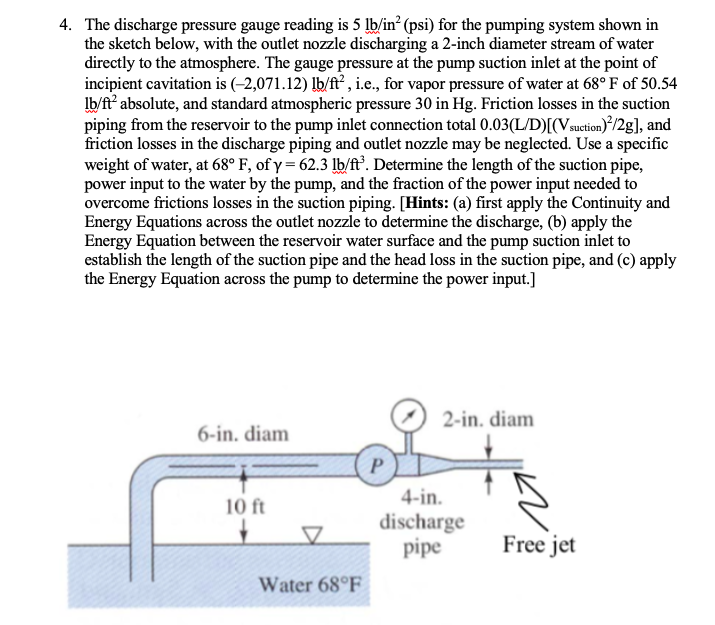4. The discharge pressure gauge reading is 5 lb/in2 (psi) for the pumping system shown in the sketch below, with the outlet nozzle discharging a 2-inch diameter stream of water directly to the atmosphere. The gauge pressure at the pump suction inlet at the point of incipient cavitation is (-2,071.12) lb/ft, i.e., for vapor pressure of water at 68° F of 50.54 lb/ft2 absolute, and standard atmospheric pressure 30 in Hg. Friction losses in the suction piping from the reservoir...

• A centrifugal pump test yields the following data: Pump Head Flow (m)(L/min) 30 25 20 40 The pump...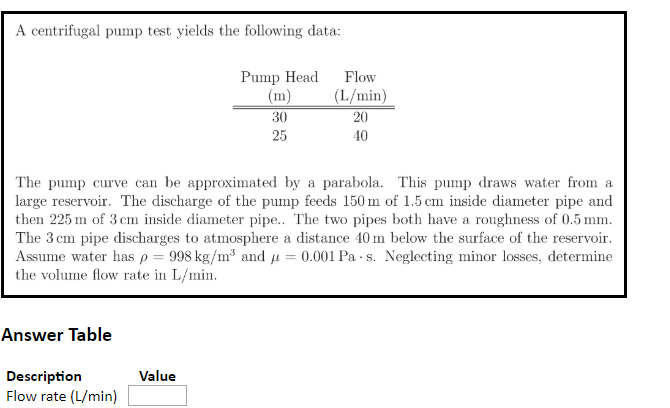A centrifugal pump test yields the following data: Pump Head Flow (m)(L/min) 30 25 20 40 The pump curve can be approximated by a parabola. This pump draws water from a large reservoir. The discharge of the pump feeds 150 m of 1.5 cm inside diameter pipe and then 225 m of 3 cm inside diameter pipe.. The two pipes both have a roughness of 0.5 mm The 3 cm pipe discharges to atmosphere a distance 40 m below the...

• Project-Design Problem: A family of water pumps produced by a given pump manufacturer have performance and...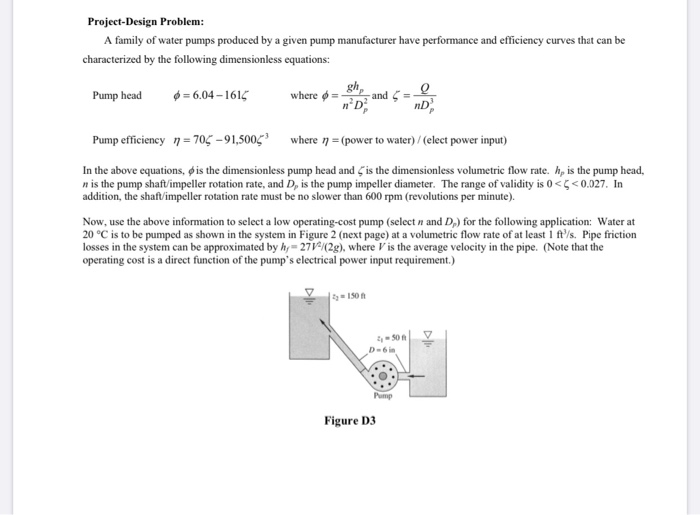Project-Design Problem: A family of water pumps produced by a given pump manufacturer have performance and efficiency curves that can be characterized by the following dimensionless equations: Pump head 6 = 6.04-1616 where and 5 nD Pump efficiency n = 705-91,5006 where n = (power to water) / (elect power input) In the above equations, is the dimensionless pump head and is the dimensionless volumetric flow rate. h, is the pump head, n is the pump shaft/impeller rotation rate, and...

• A pump delivers water at 200C between a reservoir and a water tank that is 75[ft] higher than the...

A pump delivers water at 200C between a reservoir and a water tank that is 75[ft] higher than the reservoir. The suction side of the system consists of a cast iron pipe of 1[ft]diameter with a strainer (Ks= 2.5) and four 90-degree bends at R/D= 5. The discharge side of the system includes a 1000[ft] long commercial steel pipe with 12” diameter followed by a gate valve. The NPSH required for the pump is 10[ft], and the design discharge is...

• 3· T ump delive lvers water at 60F from the lower at a rate of 2.5 ft/s. The suction line is 12-ft gs shown and the discharge line has a e the long with fittings ength of 190-ft with fittings sho...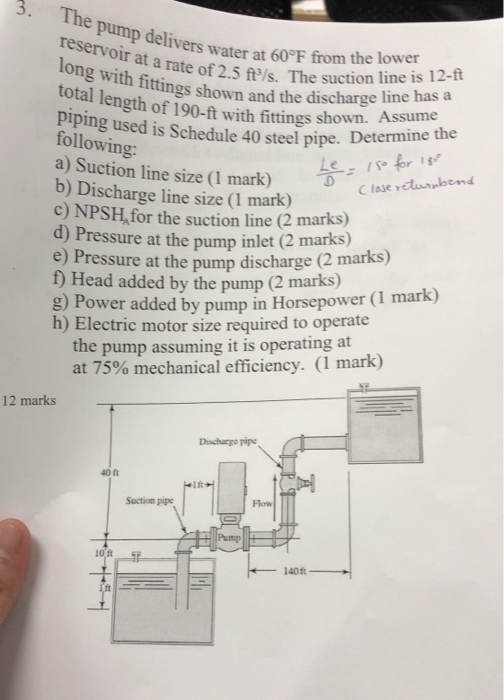3· T ump delive lvers water at 60F from the lower at a rate of 2.5 ft/s. The suction line is 12-ft gs shown and the discharge line has a e the long with fittings ength of 190-ft with fittings shown. Assume piping used is Schedule 40 steel pipe. Detc following: a) Suction line size (1 mark) /0aase returs b) Discharge line size (1 mark) c) NPSH,for the suction line (2 marks) d) Pressure at the pump inlet (2 marks)...

• 7.14 The pump in Fig. 7.20 delivers water from the lower to the upper reservoir at the rate of 0.057 m3/s. The energy loss between the suction pipe inlet and the pump is 1.83 m and that between t...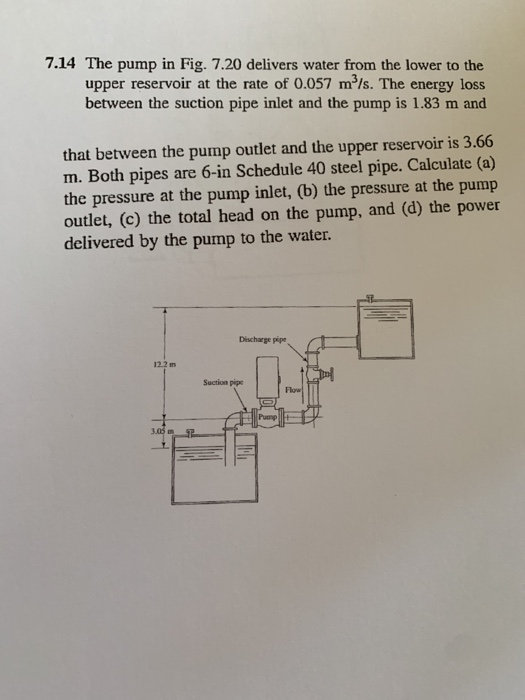7.14 The pump in Fig. 7.20 delivers water from the lower to the upper reservoir at the rate of 0.057 m3/s. The energy loss between the suction pipe inlet and the pump is 1.83 m and that between the pump outlet and the upper reservoir is 3.66 m. Both pipes are 6-in Schedule 40 steel pipe. Calculate (a) the pressure at the pump inlet, (b) the pressure at the pump outlet, (c) the total head on the pump, and (d)...

• 02. centrifugal pump draws benzene at 25°C (sp. weight of benzene 8.59 kN/m) om a tank whose level is 2.6 m below the pump inlet. The atmospheric pressure (abs). The head loss in the suction pipi...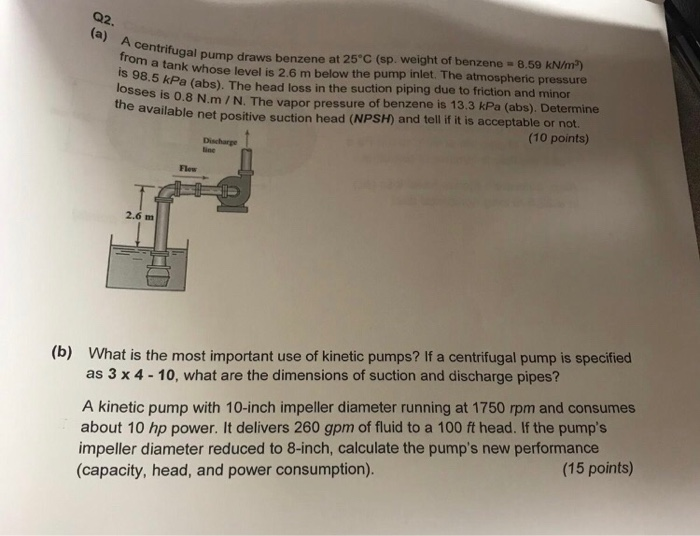02. centrifugal pump draws benzene at 25°C (sp. weight of benzene 8.59 kN/m) om a tank whose level is 2.6 m below the pump inlet. The atmospheric pressure (abs). The head loss in the suction piping due to friction and minor osses is 0.8 N.m /N. The vapor pressure of benzene is 13.3 kPa (abs). Determine he available net positive suction head (NPSH) and tell f it is acceptable or not. (10 points) Discharge ine Flew 2.6 m What is...

• A centrifugal pump is pumping water at a rate of 35.55 gal/min. The pressures at the...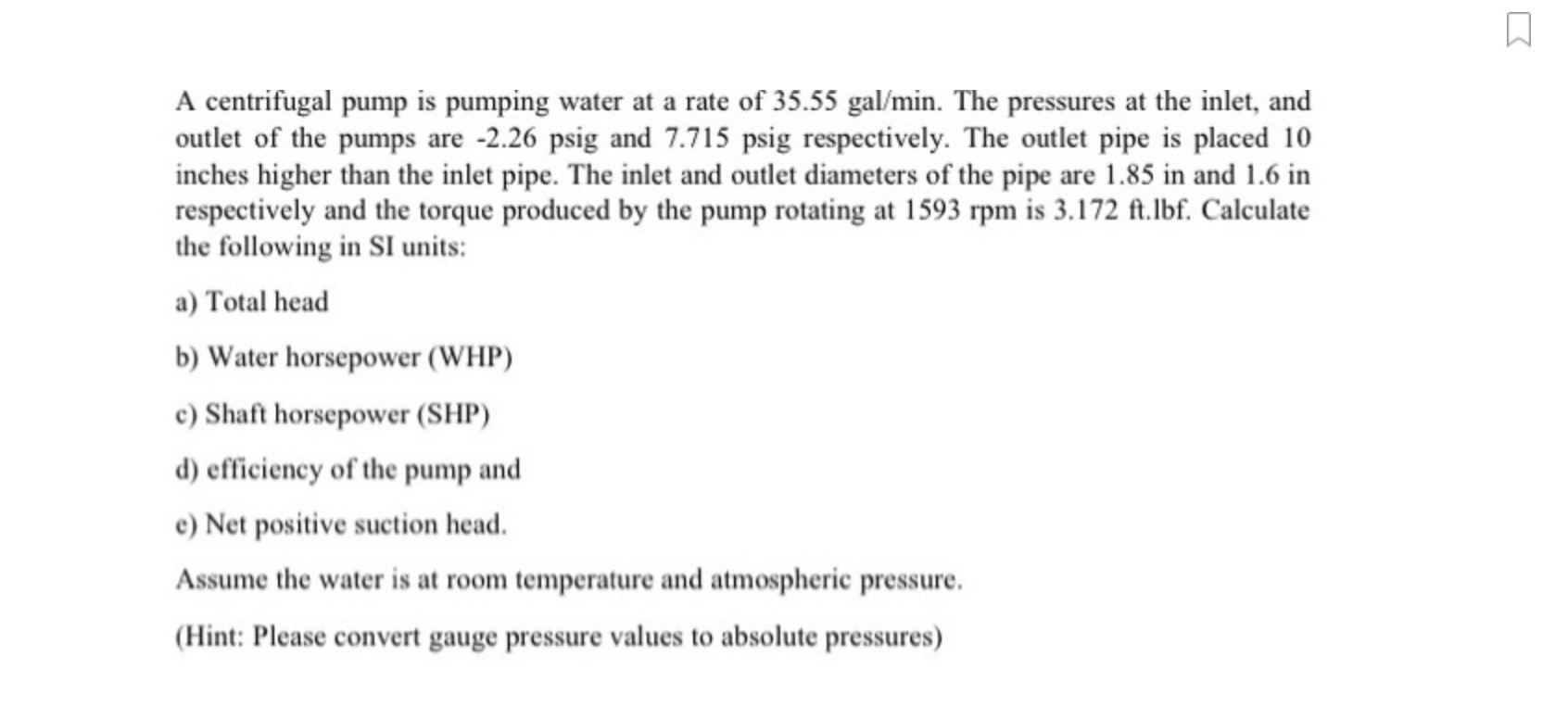A centrifugal pump is pumping water at a rate of 35.55 gal/min. The pressures at the inlet, and outlet of the pumps are -2.26 psig and 7.715 psig respectively. The outlet pipe is placed 10 inches higher than the inlet pipe. The inlet and outlet diameters of the pipe are 1.85 in and 1.6 in respectively and the torque produced by the pump rotating at 1593 rpm is 3.172 ft.Ibf. Calculate the following in SI units: a) Total head b)...